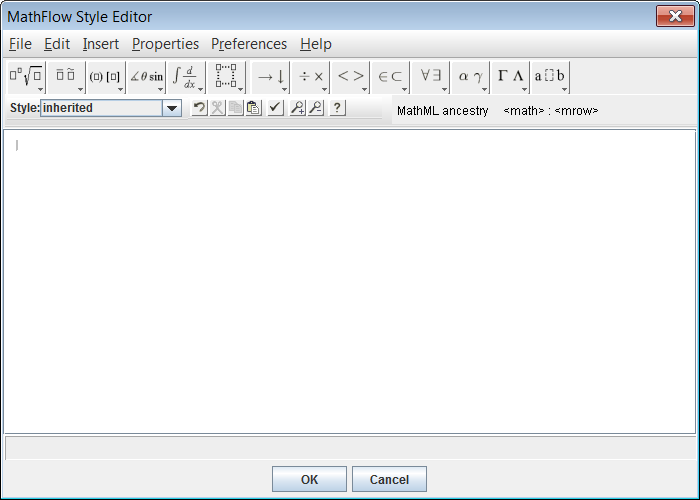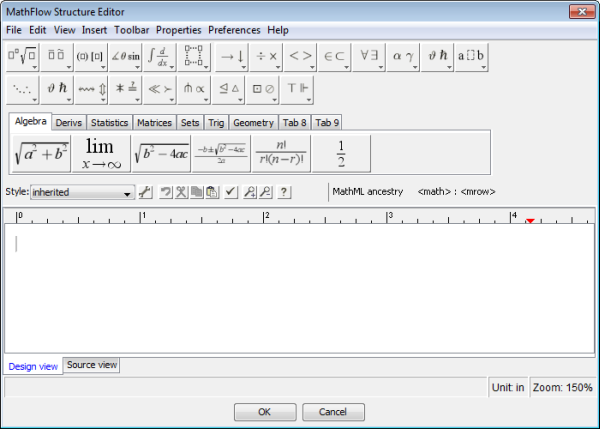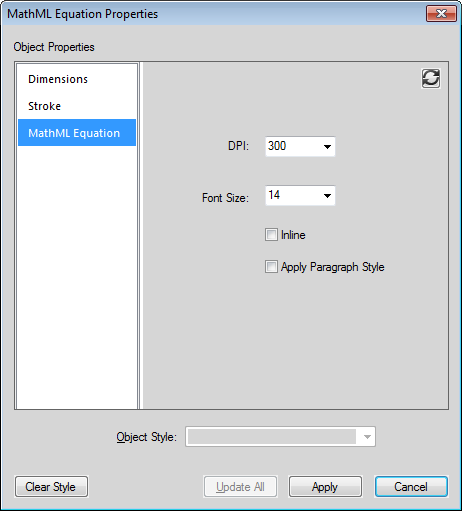### Create equations using MathML

In addition to using the FrameMaker Equation pod to add equations to your documents, you can also use the MathFlow editor from Design Science to design complex mathematical equations. You can then add these equations to your FrameMaker documents. You also have the option to later modify these equations in the same MathFlow editor and publish documents containing MathML equations.

MathML equations are available for use in FrameMaker structured and unstructured documents. These equations are also available for use in FrameMaker XML Author.

note:  You can also insert MathML equations in DITA 1.3 topics (topic, task, concept, reference, and troubleshooting.) A new element named, “mathml” is created when you insert a MathML equation.

If you generate Apply conditional tags for a document containing MathML equations, a reader can search for the contents of these equations.

FrameMaker ships with a trail version of the following MathFlow editors:

MathFlow™ Style Editor is for content authors and subject matter experts (SME). It provides precise control over the visual appearance of math expressions, a style toolbar for quickly adjusting fonts, and an easy to use interface for modifying a wide range of equation properties from operator spacing to matrices/table appearance.

Style EditorMathFlow™ Structure Editor is for XML content professionals. It provides fine control over visual presentation and its underlying MathML structure. There is a Source View enabling the user to add processing instructions and comments directly to the MathML, a ruler for precise layout of the equation, and a tabbed toolbar for storing commonly used equations or frag­ments.

Structure EditorFor a feature comparison of the editors, see MathFlow Editors. Also, for the procedure to upgrade to the full version of the MathFlow editor, see Configure the installation settings.

See the video, MathML in unstructured documents.

#### Create and insert a MathML equation into a document

1)On the Special menu, choose MathML Equation.

2)Create an equation in the MathFlow Editor window.

For details on how to create equations in the MathFlow see the MathFlow help. You can launch the MathFlow help from within the MathFlow Editor window.

3)To add the equation to the document, click OK.

The equation is inserted into the document.

note:  If you get the font initialization failed error message, see the KB article - MathML font initial­ization error for resolution.

When the MathML equation is created, an image (.PNG) file is inserted in the document.

note:  FrameMaker does not support the .EPS file format for MathML images.

#### Edit a MathML equation in a document

1)Double-click the equation in the document or right-click on the equation and choose Edit with MathFlow.

2)Edit the equation in the MathFlow Editor window.

3)To update the equation in the document, click OK.

FrameMaker also supports the copy-paste and undo-redo operation on equations on MathML equa­tions in a document.

important:  For structured (DITA 1.3) and unstructured document, you can insert MathML equa­tions at any appropriate location of the document. However, in the case of your own structured docu­ments, you will need to first define an element in the Elements catalog that supports this type of object. FrameMaker ships with a sample structured app (for DITA 1.2) that includes a MathML element. For details how to use this element, see Sample DITA MathML structured app.

#### Configure the MathFlow settings in FrameMaker

To configure MathML settings in FrameMaker, open the Preferences dialog and go to the MathML tab.

#### Configure the installation settings

FrameMaker ships with a 30 day trial version of Style and Structure editor of MathFlow from Design Science. You can obtain the full version of the Style or Structure editor from Design Science and inte­grate that with FrameMaker.

1)After you have installed the full version of the Style or Structure editor, go to the MathFlow section of the MathML tab.

2)The trial version that ships with FrameMaker is installed in the Adobe FrameMaker installa­tion path. If you have installed MathFlow in an alternative path, specify that path.

3)In the License File Path text box, specify the path to the license file and click OK.

You need to restart FrameMaker to ensure these changes take effect.

note:  The MathFlow 30 day trial period starts from the first time to invoke the editor. Not from the day you install the version of FrameMaker that includes the editor.

#### Format a MathML equation

You can update the formatting of a selected MathML equation in a document or you can change the preferences for all MathML equations. By default, the font size of the equations is set to 14 px. DPI for the images that FrameMaker inserts into a document for each equation defaults to 300 dpi. Also, you can choose to place an equation inline with the enclosing paragraph and you can apply the formatting of the enclosing paragraph to the equation.

To update the formatting of the current selected MathML equation:

1)Select the MathML equation and choose Graphic > Object Properties.

Or choose Graphic > Object Style Designer.

These options are also available in the right-click menu.

2)Update the formatting for the current selected MathML equation in the MathML Equation Properties dialog.

MathML Equation Properties dialogDPI and Font

Change the DPI and Font size settings.

Inline

Place the image inline with the enclosing paragraph.

Apply Paragraph Style

Apply the following formats of the enclosing paragraph to the equation:

Font

Font family

Background color

Foreground color

You can also apply these settings globally to all MathML equations created subsequently.

1)Open the Preferences dialog (Edit > Preferences).

2)In the MathFlow settings section of the MathML tab, change the equation settings.

These settings take effect immediately. So you do not need to restart FrameMaker.

#### Configure the MathFlow editor

The trial version of the MathFlow editor includes the Style and Structure editors. During this period, you can choose between either of these editors.

1)To change the MathFlow editor, go to the Editor Type section of the MathML tab.

2)Choose the required MathFlow editor and click OK.

You need to restart FrameMaker to ensure these changes take effect.

note:  When installing the full version of MathFlow, you need to choose between the Style and Struc­ture editors. The MathFlow trial integration with FrameMaker includes both the editors. So you are recommended to use both these editors when trying out this feature.

#### Sample DITA MathML structured app

FrameMaker includes a sample DITA 1.2 MathML application named DITA_1.2_MathML_Sample. This mathml element has complete support for the MathML equations that are rendered by the MathFlow Style and Structure editors. To add MathML to a docu­ment, you can create a file based on this application and then use the MathML element in it.

See the video, MathML in structured documents.

##### Insert a MathML type element

1)From the File menu, choose New -> XML.

2)In the New XML dialog, go to the Structured Applications tab and choose DITA_1.2_MathM­L_Sample and click OK.

A new document is created.

3)Go to any part of the document where you want to insert a MathML equation.

The MathML element, mathml, is available in the Elements catalog of the sample structured app.

note:  A mathml element is available at any point in the document where a foreign element is available.

4)Double-click the mathml element to insert it at the point in the document.

5)Go to the XML view of the current document.

The XML of the structured document contains a MathML node at the location where you inserted the MathML element.

note:  Each element within the mathml node has an mml prefix. This prefix is used to avoid name conflicts with other elements used in the XML either from the Elements catalog of the struc­tured app or from elements defined in the MathML structure.

You also have the option to edit the equation within the mathml node in the XML view. The changes can then be seen in the WSIYWIG view.